# Computer-Based Signal Acquisition

Edition Date: June 2018
Part Number: 370858P-01
View Product InfoDIAdem 2017 HelpDIAdem 2018 HelpDIAdem 2019 HelpDIAdem 2020 HelpDIAdem 2020 SP1 Help

The following figure shows the basic processes involved in the acquisition of temperature measurement values with DIAdem DAC. The top display solves the task with a data acquisition measurement plugin, and the bottom display solves the task with an external measurement device connected to the computer via a digital interface such as GPIB or USB.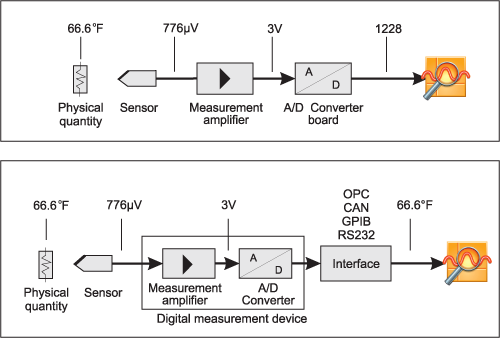Temperature Measurement Using a Plug-In Board or an Interface

For measured value acquisition, sensors first convert the physical quantities, which are to be measured, into voltage or current. This creates, for example, a temperature-related voltage at a thermocouple. The sensor signals are then transferred to a measurement amplifier and converted into numeric values for the computer, using an A/D converter. The A/D converter converts the continuous, analog signal into measured values that are time-discrete and amplitude-discrete. DIAdem can reconvert the measured values into the original physical quantities or save the values for later offline analyses.

## Communication with Measurement Hardware

DIAdem DAC communicates with the measurement hardware via the DIAdem drivers, which are available for many different kinds of measurement hardware. If no driver is available for a specific measurement hardware, you can use a DLL-based driver interface to create and implement the driver for DIAdem yourself.

In most cases, DIAdem automatically provides the capabilities of the supported hardware when you load the device driver. You only need to specify the settings in the configuration dialog box to specify the base settings for the measurement hardware.

Refer to Registering Hardware and Communicating via Interfaces for more information on how to register hardware in DIAdem or how to access external devices via interfaces.

## Parameters for A/D Conversion

The following sections provide a rough overview of the most important factors which impact the digitalized signals.

### Measurement Mode

If a measurement system is unilaterally grounded, all inputs are connected to one grounding point, which means single ended. Preferably use this system for voltages <1 Volt, for short signal cables (<4 m), and if all the input channels have a common grounding (single-ended).

In all other cases, it is better to use a differential measurement system, where each input has its own reference potential. A differential system basically has two important advantages compared to a single-ended system: Ground loops cannot cause measurement errors, and most interferences that affect both measurement cables of a signal are, for the most part, suppressed. On the other hand, unilaterally grounded systems have twice as many measurement channels.

### Resolution

An analog signal is digitized into a discrete signal. The amplitude of the signal is represented in discrete steps, which results in a digitization error called the quantization error.

The number of bits the A/D converter uses determines the number of steps the analog signal is digitized with. With the increasing number of steps the resolution improves, and the quantization error and the smallest measurable signal change decrease. A 3-bit A/D converter, for example, divides its input voltage range into 23 = 8 steps. A binary or digital code between 000 and 111 represents the eight subsections.

The following figure shows a 5 kHz sine oscillation digitized by a 3-bit A/D converter. The digital signal does not adequately represent the original signal, because the converter does not have enough digital subsections. If you increase the resolution to 16 bits in order to increase the number of subsections in the A/D converter from 8 (23) to 65536 (216), you achieve a precise representation of the analog signal.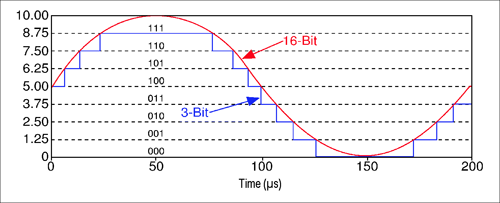Resolution of a 3-Bit and a 16-Bit A/D Converter

### Measurement Range and Gain

The measurement range refers to the minimum and the maximum analog signal levels that the A/D converter can digitize. Many devices have selectable measurement ranges (usually from 0 to 10 Volt or from –10 to +10 Volt), so you can adjust the range of the A/D converter to the signal range. This best exploits the available resolution for an exact signal measurement.

In the following figure, the 3-bit A/D converter in the left diagram (1) has 8 digital subsections in the measurement range from 0 to 10 Volt. However, if you select the measurement range –10 to +10 Volt, as shown in diagram (2), the same A/D converter divides the 20 V measurement range into eight subsections. The lowest measurable voltage change increases from 1.25 to 2.5 Volts, which means the right diagram displays the signal considerably less precisely.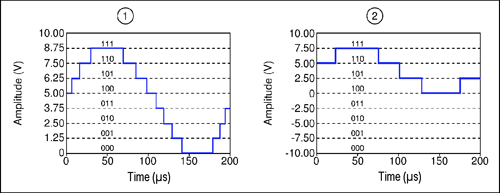How the Measurement Range Affects Precision: (1) 0 to 10 Volt, (2) –10 to +10 Volt

The amplification factor includes all amplifications and attenuations that affect a signal before the signal is digitized. If you adjust the amplification factor to the signal level, you make the best use of the resolution of an A/D converter. Therefore, it is just as important to set the correct amplification as it is to set the right measurement range.

### Sampling Rate

We have shown that digital signals have discrete steps to display the amplitudes, and we have described how to minimize the resulting quantization errors. However, we have not taken into account the fact that after A/D conversion, the signal is no longer continuous along the time axis. This is due to the fact that the signal is sampled only at specific times, and the samples are stored as numbers. Random sampling never gives an exact representation of the data.

The more frequent the samples, the more precisely you can reconstruct the original analog signal as a series of digital numeric values. However, this greatly increases the required sampling rate, especially for high-frequency signals, which in turn results in increasingly large amounts of data per unit of time.

This correlation results in the following requirement: you need a sampling rate that meets the requirements with as few samples as possible.

According to the Nyquist theory, the sampling rate should be at least twice as high as the highest frequency in the signal. The digitized signal then contains enough information to resolve, for example, the frequencies of the acquired signal after a transformation to the frequency domain (FFT). However, as shown in the following figure, this does not in any way provide an exact reconstruction of the signal profile. Neither the exact signal form nor the amplitude heights can be reconstructed for all points in time.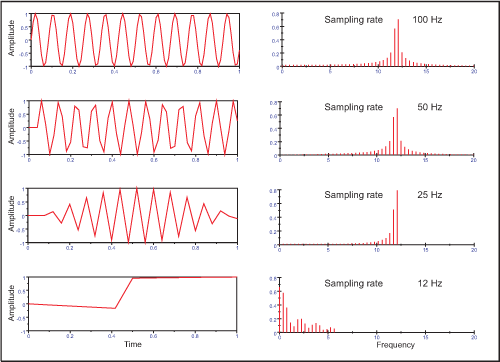How a Low Sampling Rate Affects Data Acquisition

As this figure shows, a multiple of the Nyquist frequency is necessary if you want to display the correct shape of the signal as well as the frequency.

### Anti-Aliasing Filter

If the sampling rate is lower than the sampling rate determined according to the Nyquist theory, an effect called Aliasing occurs. This effect causes digitized signal forms or signal frequency parts that are essentially different from the actual analog signal (alias frequencies). The data generated by undersampling display frequency contents that are substantially lower than the original signal, as shown in two examples in the following figure.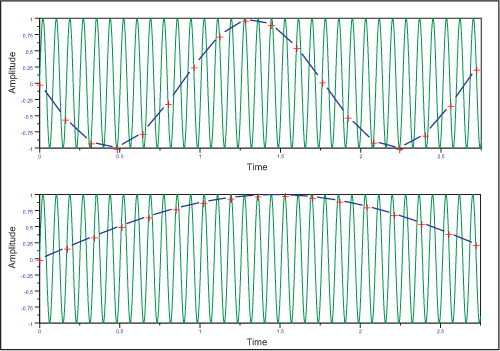Aliasing Effects Caused by Undersampling a Signal

You cannot distinguish between these apparent signal sections of the digitized signal and the real signal frequencies. Therefore, you cannot correct errors caused by aliasing.

If you cannot attain the sampling frequency that is required to measure a signal, or if the highest frequency in the signal is unknown, you must filter the analog signal with lowpass filters (anti-aliasing filters) before the A/D conversion. Alternatively, you can increase the sampling frequency by using other measurement hardware or a different type of measurement.

According to the Nyquist criterion, an appropriate lowpass filter must only let through signals that have frequencies lower than half the sampling rate used for the A/D conversion. In this case, filters that have very steep slopes are most suitable.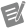Note  Do not confuse anti-aliasing filters with digital filters on the digital side after the A/D converter.

Unwanted noise distorts the analog signal before the signal is converted into a digital signal. You can limit external influences by using suitable signal conditioning, such as filtering and amplification, before digitization. Another way to reduce noise distortion is to oversample the signal, which means using a higher sampling rate for data acquisition and then averaging the digitized values.

# Computer-Based Signal Output

With the right hardware, DIAdem can output acquired data, generated data, and calculated data as voltage or current. You can use analog or digital outputs to control processes with DIAdem, or to create closed loops with control blocks.

The following figure shows the signal output for temperature control. DIAdem converts the actuating variable, which is determined by comparing the room temperature and the set point, into the manipulated variable for the heating valve. DIAdem transfers a numeric value to the D/A converter, on a plug-in board for example, which outputs the proportional voltage. A power stage transfers the voltage to the heating valve. Assuming the set temperature is 20 °C, the heating valve opens wider and releases more hot water into the heating system.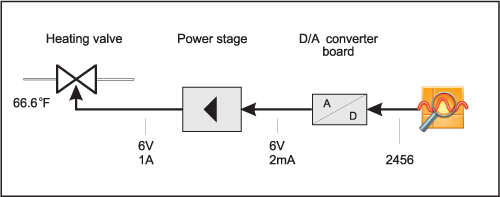Temperature Control with a Plug-In Board